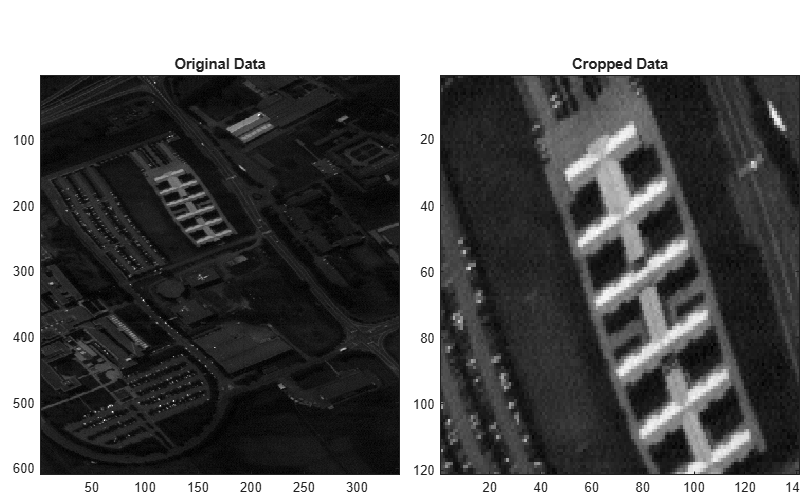# cropData

Crop regions-of-interest

## Syntax

``newhcube = cropData(hcube,row,column)``
``newhcube = cropData(hcube,row,column,band)``

## Description

````newhcube = cropData(hcube,row,column)` crops the regions of interest (ROIs), specified by `row` and `column`, across all the spectral bands in the hyperspectral data cube `hcube`. The function returns the cropped data as a new `hypercube` object `newhcube`.```

example

````newhcube = cropData(hcube,row,column,band)` crops the ROIs across the specified spectral bands `band`. NoteThis function requires the Image Processing Toolbox™ Hyperspectral Imaging Library. You can install the Image Processing Toolbox Hyperspectral Imaging Library from Add-On Explorer. For more information about installing add-ons, see Get and Manage Add-Ons. ```

## Examples

collapse all

Read hyperspectral data from an ENVI format file.

`hcube = hypercube('paviaU.dat');`

Crop the first 10 spectral bands of the input data cube.

`newhcube = cropData(hcube,':',':',1:10);`

Specify the row and column indices of the ROI to crop from the extracted bands.

```row = 130:250; column = 60:200;```

Crop the ROI.

`newhcube = cropData(newhcube,row,column,':');`

Display both bands in the original and the cropped versions of a spectral band.

```fig = figure('Position',[0 0 800 500]); axes1 = axes('Parent',fig,'Position',[0.05 0.05 0.45 0.8]); imagesc(hcube.DataCube(:,:,5),'Parent',axes1) title('Original Data') axes2 = axes('Parent',fig,'Position',[0.55 0.05 0.45 0.8]); imagesc(newhcube.DataCube(:,:,5),'Parent',axes2) title('Cropped Data') colormap gray```## Input Arguments

collapse all

Input hyperspectral data, specified as a `hypercube` object. The `DataCube` property of the `hypercube` object contains the hyperspectral data cube.

Row indices of the data cube, specified as `':'`, a positive integer, or a vector of positive integers.

• To select all the rows in the data cube, use ` ':'`.

• To select a particular row or rows, specify the row index as a positive integer or vector of positive integers respectively. If the data cube is of size M-by-N-by-C, the specified row index values must all be less than or equal to M. To specify a range of row indices, or indices at a regular interval, use the `colon` operator. For example, `row = 1:10`.

Data Types: `single` | `double` | `int8` | `int16` | `int32` | `int64` | `uint8` | `uint16` | `uint32` | `uint64` | `char` | `string`

Column indices of the data cube, specified as `':'`, a positive integer, or a vector of positive integers.

• To select all the columns in the data cube, use ``` ':'```.

• To select a particular column or columns, specify the column index as a positive integer or vector of positive integers respectively. If the data cube is of size M-by-N-by-C, the specified column index values must all be less than or equal to N. To specify a range of column indices, or indices at a regular interval, use the `colon` operator. For example, ```column = 1:10```.

Data Types: `single` | `double` | `int8` | `int16` | `int32` | `int64` | `uint8` | `uint16` | `uint32` | `uint64` | `char` | `string`

Spectral band numbers, specified as `':'`, a positive integer or a vector of positive integers.

• To select all the bands in the data cube, use `':'`.

• To select a particular band or bands, specify the band number as a positive integer or vector of positive integers respectively. If the data cube is of size M-by-N-by-C, the specified band number values must all be less than or equal to C. To specify a range of band numbers or numbers at a regular interval, use the `colon` operator. For example, ```band = 1:10```.

Data Types: `single` | `double` | `int8` | `int16` | `int32` | `int64` | `uint8` | `uint16` | `uint32` | `uint64` | `char` | `string`

## Output Arguments

collapse all

Output hyperspectral data, returned as a `hypercube` object.